# Decimals on a Number Line: Counting in Tenths

Decimals on a Number Line: Counting in Tenths• Here is a number line between zero and one.
• There are 10 lines dividing the total distance into 10 equal parts.
• One whole divided into ten equal parts is   1 / 10  .
•   1 / 10   as a decimal is 0.1.
• At each new line, we are adding 0.1 or one tenth.
• To count in tenths, the digit after the decimal point increases by one each time.
• We have 0.1, 0. 2, 0.3, 0.4, 0.5, 0.6, 0.7, 0.8 and 0.9.
• The line representing 0.5 is slightly thicker than the other lines to show that it is half way between 0 and 1

• Ten tenths is equal to one whole.
Dividing a whole number into ten equal parts makes tenths or 0.1.

We add 0.1 at each new increment.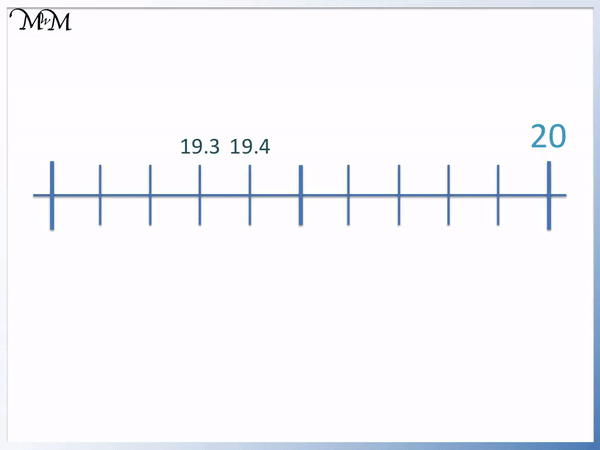• There are ten equal parts between 19 and 20 and so each increment is one tenth.
• Moving from one line to the next is moving by one tenth or 0.1.
• We can count backwards from 19.3 to get to 19.2, 19.1 and then 19.
• We can count up from 19.4 to get to 19.5, 19.6, 19.7, 19.8 and 19.9.
• The two decimal numbers indicated by the arrows are 19.2 and 19.8# Counting in Tenths with Decimals on a Number Line

In this lesson, we will look at placing decimals on a number line. Specifically we will be counting in tenths on a number line.

In the animation below, we have a number line ranging from zero to one. To get from zero to one, there are ten equal steps, marked with a line.

We have divided a whole number into 10 parts. This means that we will be counting up in tenths, or 0.1s.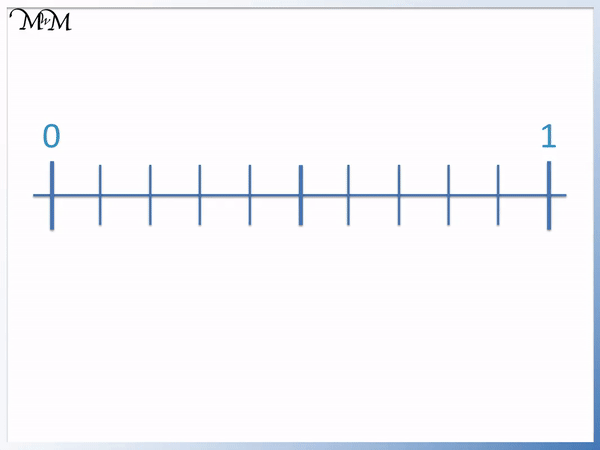The first line on the scale corresponds to an increment of 0.1, which is the same as one tenth.

The second line is 0.2, which is two tenths.

The third line is 0.3, which is three tenths.

The fourth line is 0.4, which is four tenths.

The fifth line is 0.5, which is five tenths. This thicker line indicates that it is the halfway point between 0 and 1.

The sixth line is 0.6, which is six tenths.

The seventh line is 0.7, which is seven tenths.

The eighth line is 0.8, which is eight tenths.

The ninth line is 0.9, which is nine tenths.

The tenth line is 1, which is the same as ten tenths, or one whole.

We can see that to move from one line to the line to its right, we simply add 1 to the digit after the decimal point.

In the following animation, we will look at the decimal numbers between one and two on a number line. The following scale is the same as the previous, with ten equal increments.

Therefore, we will be counting in tenths, or 0.1s.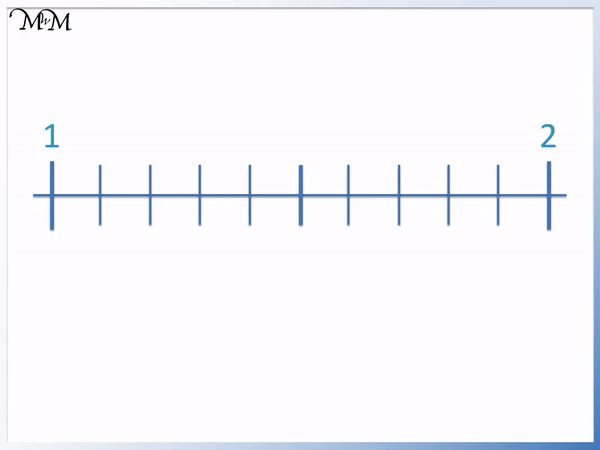1 is the same as 1.0 but we simply write 1.

2 is the same as 2.0 but we simply write 2.

Starting from 1, we have 1.1, 1.2, 1.3, 1.4, 1.5, 1.6, 1.7, 1.8, 1.9 and 2.

We increase the digit after the decimal point by 1 at each new line.

It can be easy to visualise this in a similar way to counting past ten in whole numbers. If we ignore the decimal point, it is like counting 11, 12, 13, 14, 15 etc.

The thicker line, labelled as 1.5, indicates the halfway point between 1 and 2.

In the example below, we are asked to label the numbers on the scale that are indicated by the arrows. The scale ranges from two to three. We have the same number of increments, so we will be counting in tenths, or 0.1s.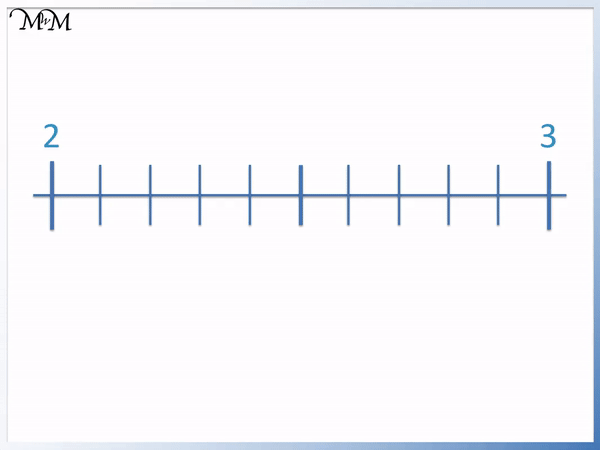To find the valued of the decimals marked on the number line with arrows, we can begin by counting up in 0.1s.

Starting from 2, we have 2.1, 2.2, 2.3 and 2.4. Our first answer is 2.4.

Continuing to count in 0.1s, we have 2.5, 2.6 and 2.7.

In the example below, we have another scale with ten increments. We will therefore be counting up in 0.1s (tenths) to find the values of the marks indicated by the arrows.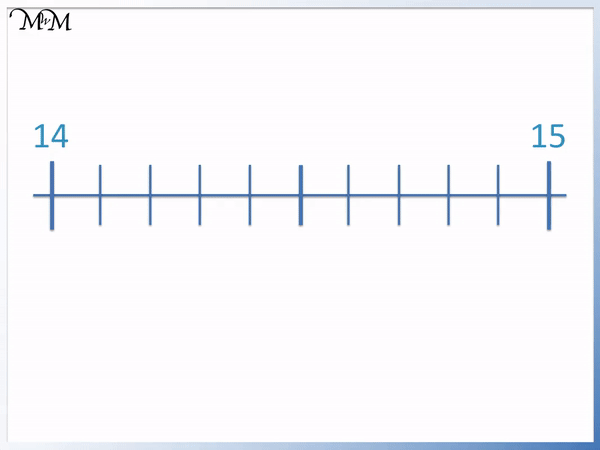Starting from 14, we have 14.1 and 14.2, which is our first answer.

Continuing from 14.2, we have 14.3, 14.4, 14.5, 14.6, 14.7, 14.8 and 14.9, which is our second answer.

We could have also found 14.9 by counting back from 15.

In our final example below, we are not given the number on the left-hand-side of the scale. However, looking at the scale, there are still ten steps to get to the number on the right-hands-side, which is 20.

We are also given the values 19.3 and 19.4. We therefore know that the increments are tenths, so we will be counting in 0.1s.If we count backwards from 19.3, we have 19.2, which is our first answer. We can continue to count backwards, and we have 19.1 and 19.

Next, we can count on from 19.4. we have 19.5, 19.6, 19.7 and 19.8.

To get 19.8, we could have also counted back from 20 (20, 19.9, 19.8).Now try our lesson on Rounding Decimals to the Nearest Whole Number where we learn what it means to round a decimal number to the nearest whole number.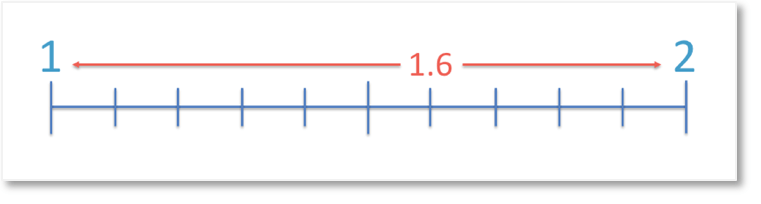error: Content is protected !!